Like   Tweet   Pin   +1   in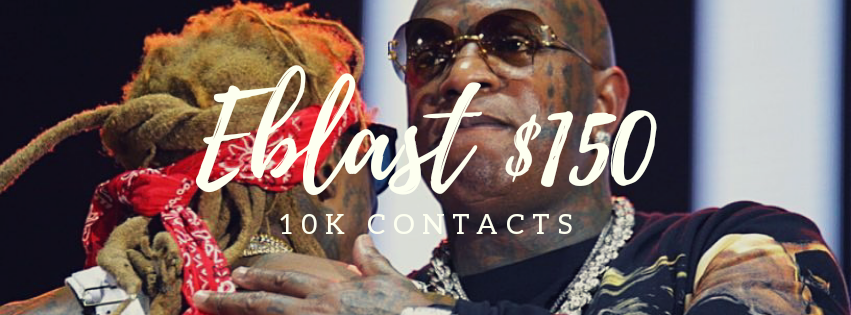Entry Level Marketing Campaign

Email Blast to 5,000 contacts \$150.00

Guaranteed indie blog placements

Guaranteed Overall Awareness of artist release

Upgrade to a Weekly EBlast Package \$350
(your blast will be sent out once a week for 1 month.)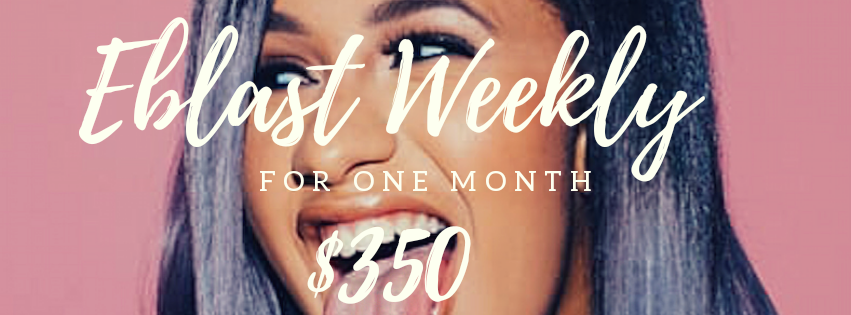Upgrade to a Weekly EBlast Package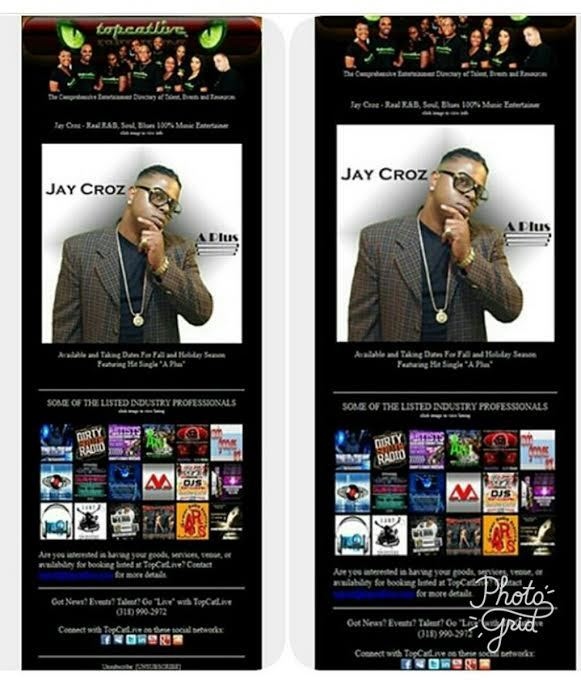Your Music Permanently Placed on our blog (Viewed by industry A&Rs from Universal, Hustle Gang, Roc- Nation, Def Jam, Capitol, Interscope, Island, Electra etc.)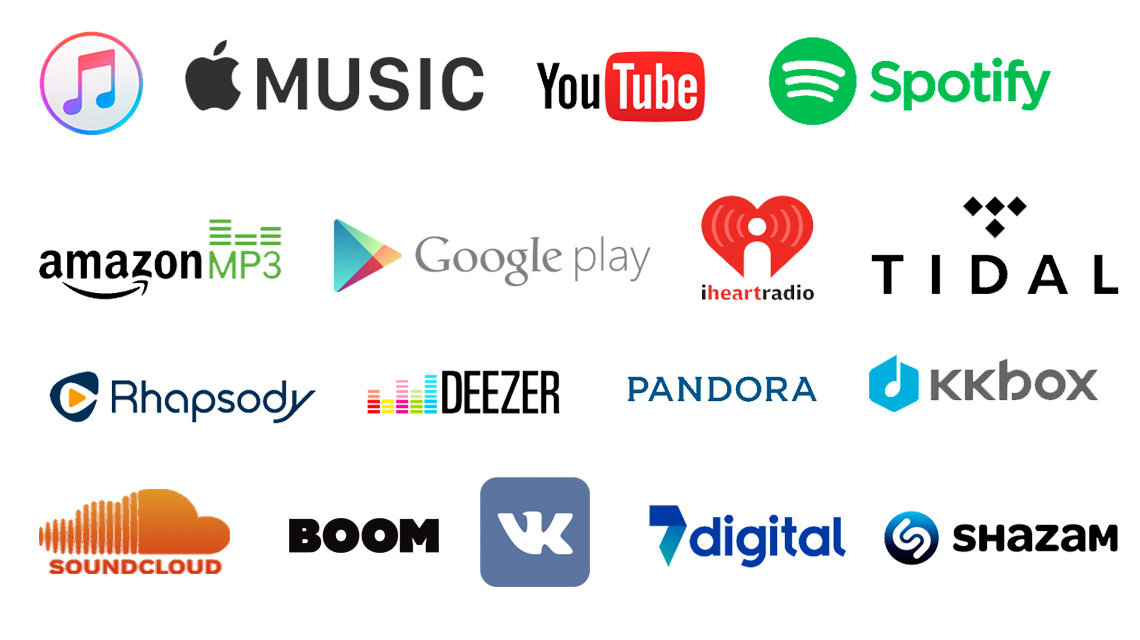We'll get your music into iTunes, Spotify, Apple Music, Pandora, Amazon, Google Play, Tidal, YouTube, Deezer and 150+ other stores & streaming services & you keep 100% of the royalties!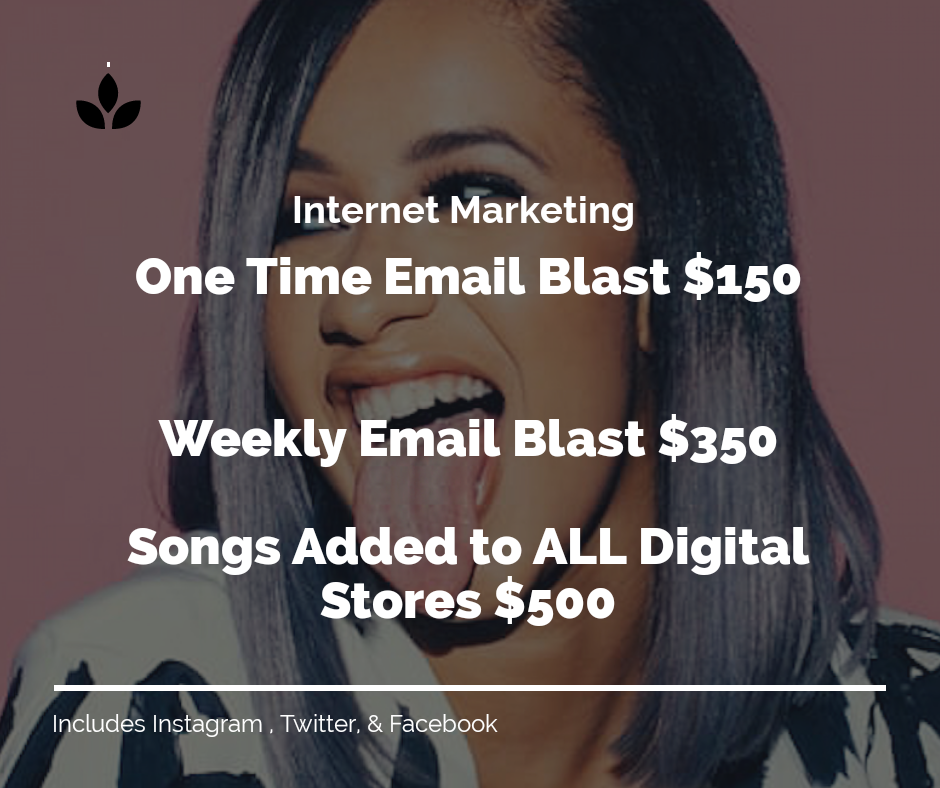## Choose payment option below to get started now!

 table div table+table+table+table+table+table+table+table+table div table{width:100%;padding:0}table div table+table+table+table+table+table+table+table+table div table table{padding:0;float:left!important;width:38.301%!important}table div table+table+table+table+table+table+table+table+table div table table+table td,table div table+table+table+table+table+table+table+table+table div table td{padding-left:0;padding-right:0}table div table+table+table+table+table+table+table+table+table div table table td{padding-left:0;padding-right:20px}table div table+table+table+table+table+table+table+table+table div table table+table{float:left!important;width:61.699%!important}/* styles */

## ACTIVE HAND 2 HAND promoHand deliver your media with Video Proof! Including but not limited to Djs, Club Promoters, & More (\$1,000)

 /* styles */ Dont forget everything above is ALSO included in this package. Upgrade Hand 2 Hand Promo PLUS include shows (\$1,500)# 6Month All-In Package \$2,500

## Choose payment option below to get started now!

 table div table+table+table+table+table+table+table+table+table+table+table+table+table+table+table div table{width:100%;padding:0}table div table+table+table+table+table+table+table+table+table+table+table+table+table+table+table div table table{padding:0;float:left!important;width:38.301%!important}table div table+table+table+table+table+table+table+table+table+table+table+table+table+table+table div table table+table td,table div table+table+table+table+table+table+table+table+table+table+table+table+table+table+table div table td{padding-left:0;padding-right:0}table div table+table+table+table+table+table+table+table+table+table+table+table+table+table+table div table table td{padding-left:0;padding-right:20px}table div table+table+table+table+table+table+table+table+table+table+table+table+table+table+table div table table+table{float:left!important;width:61.699%!important}/* styles */

## Want to be featured on StripperRadio...over 7million plays on Soundcloud to date!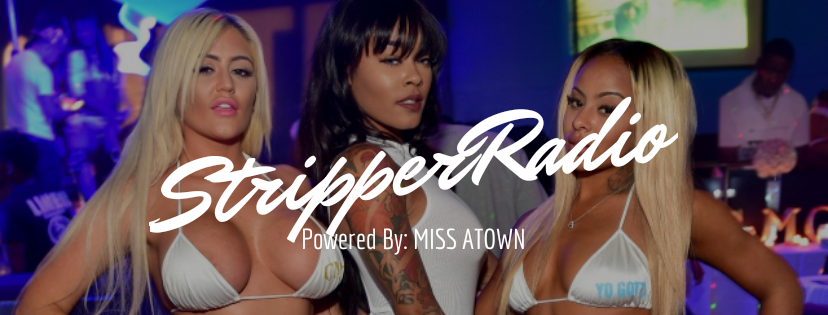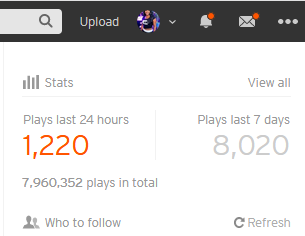table div table+table+table+table+table+table+table+table+table+table+table+table+table+table+table+table+table+table div table,table.module-17{width:71.7%;float:left;padding:0}table div table+table+table+table+table+table+table+table+table+table+table+table+table+table+table+table+table+table div table a{border:0 none;text-decoration:none}table div table+table+table+table+table+table+table+table+table+table+table+table+table+table+table+table+table+table div table img{width:100%!important;border:0 none;text-decoration:none}table div table+table+table+table+table+table+table+table+table+table+table+table+table+table+table+table+table+table div table td{width:100%;padding:0 20px 20px 0}/* styles */ \$50 gets you added to our Soundcloud playlist!

## Choose payment option below to get started now!

 table div table+table+table+table+table+table+table+table+table+table+table+table+table+table+table+table+table+table+table+table div table{width:100%;padding:0}table div table+table+table+table+table+table+table+table+table+table+table+table+table+table+table+table+table+table+table+table div table table{padding:0;float:left!important;width:38.301%!important}table div table+table+table+table+table+table+table+table+table+table+table+table+table+table+table+table+table+table+table+table div table table+table td,table div table+table+table+table+table+table+table+table+table+table+table+table+table+table+table+table+table+table+table+table div table td{padding-left:0;padding-right:0}table div table+table+table+table+table+table+table+table+table+table+table+table+table+table+table+table+table+table+table+table div table table td{padding-left:0;padding-right:20px}table div table+table+table+table+table+table+table+table+table+table+table+table+table+table+table+table+table+table+table+table div table table+table{float:left!important;width:61.699%!important}/* styles */

## Mixtape SlotsMixtape Slots And Hosting!We offer mixtape slots for your singles and we offer full mixtape hosting packages!
Slots starting at \$50 | Hosting starting at \$500. Placed on all mixtape streaming platforms; such as, but not limited to, MyMixtapez, Spinrilla, LiveMixtapes, Datpiff.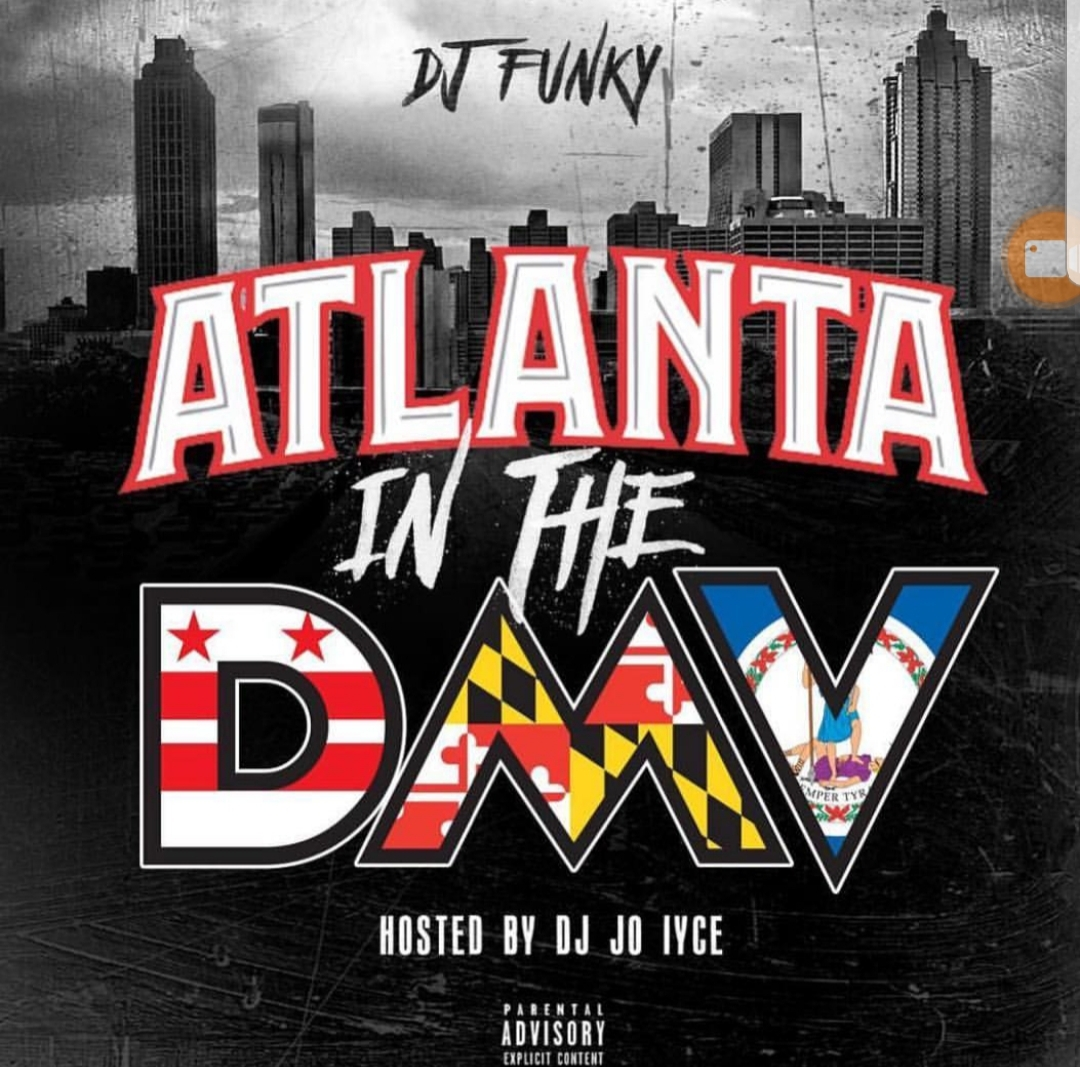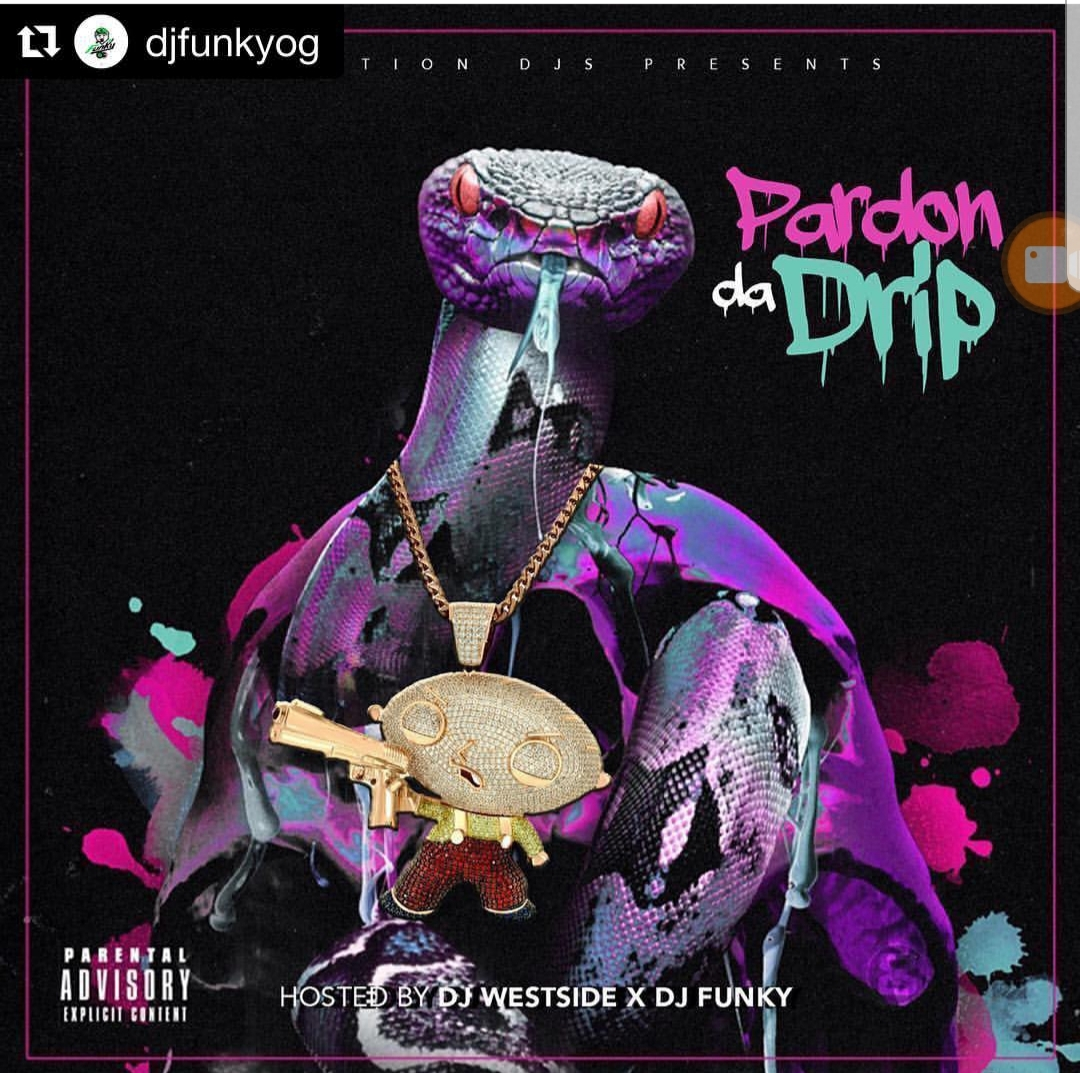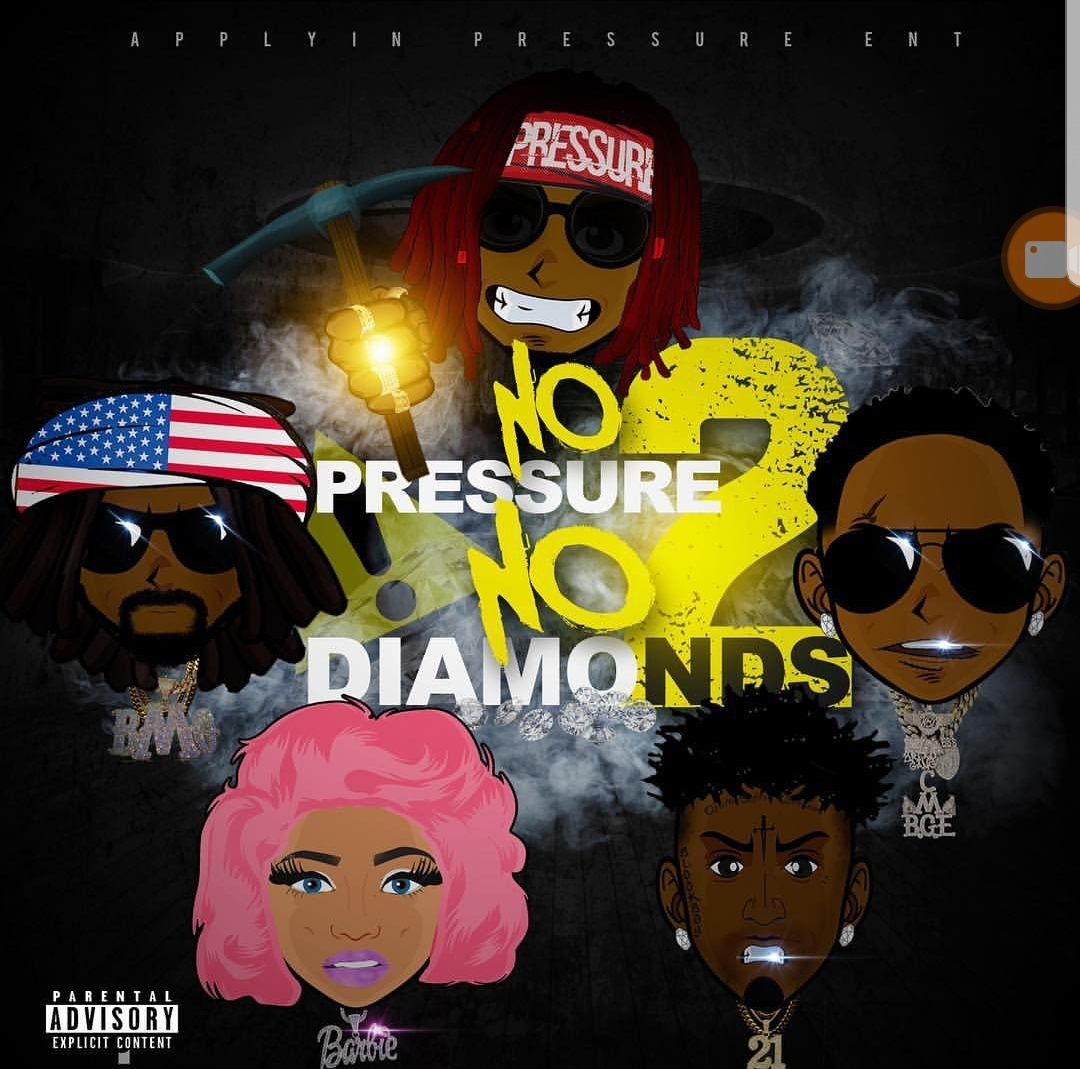## Choose payment option below to get started now!

 table div table+table+table+table+table+table+table+table+table+table+table+table+table+table+table+table+table+table+table+table+table+table+table+table+table div table{width:100%;padding:0}table div table+table+table+table+table+table+table+table+table+table+table+table+table+table+table+table+table+table+table+table+table+table+table+table+table div table img{width:96.23%;padding:0;float:none}table div table+table+table+table+table+table+table+table+table+table+table+table+table+table+table+table+table+table+table+table+table+table+table+table+table div table td{width:100%;padding:0 1.88% 18px}/* styles */table div table+table+table+table+table+table+table+table+table+table+table+table+table+table+table+table+table+table+table+table+table+table+table+table+table+table div table{width:100%;padding:0}table div table+table+table+table+table+table+table+table+table+table+table+table+table+table+table+table+table+table+table+table+table+table+table+table+table+table div table table{padding:0;float:left!important;width:38.301%!important}table div table+table+table+table+table+table+table+table+table+table+table+table+table+table+table+table+table+table+table+table+table+table+table+table+table+table div table table+table td,table div table+table+table+table+table+table+table+table+table+table+table+table+table+table+table+table+table+table+table+table+table+table+table+table+table+table div table td{padding-left:0;padding-right:0}table div table+table+table+table+table+table+table+table+table+table+table+table+table+table+table+table+table+table+table+table+table+table+table+table+table+table div table table td{padding-left:0;padding-right:20px}table div table+table+table+table+table+table+table+table+table+table+table+table+table+table+table+table+table+table+table+table+table+table+table+table+table+table div table table+table{float:left!important;width:61.699%!important}/* styles */

## Learn About Our Affiliates and Afilliate Service Plus Miss Atown Media Services

 /* styles */ In the Music Industry as in with any business, your NETWORK is your NETWORTH and Miss Atown has an extensive network to help you reach as high as you want to go. Miss Atown is also a media queen and offers various services in those areas. Click Here to find out more.
 /* styles */

## Come out and be apart of the 8th Annual Atlanta Hip-Hop Day, October 6th and 7th.

 /* styles */ To find out more and to sign up to be a part of the 8th Annual Atlanta Hip-Hop Day | Click Here
 table div table+table+table+table+table+table+table+table+table+table+table+table+table+table+table+table+table+table+table+table+table+table+table+table+table+table+table+table+table+table+table+table div table{width:100%;padding:0}table div table+table+table+table+table+table+table+table+table+table+table+table+table+table+table+table+table+table+table+table+table+table+table+table+table+table+table+table+table+table+table+table div table img{width:96.23%;padding:0;float:none}table div table+table+table+table+table+table+table+table+table+table+table+table+table+table+table+table+table+table+table+table+table+table+table+table+table+table+table+table+table+table+table+table div table td{width:100%;padding:0 1.88% 18px}/* styles */Connect w/ Miss Atown on Social Media: